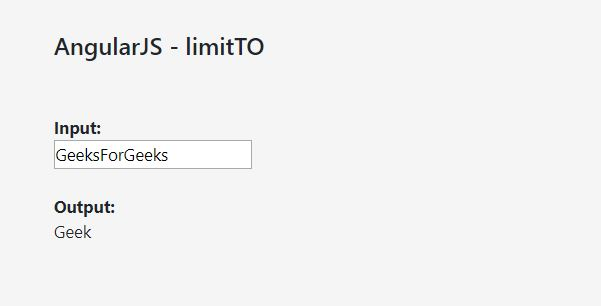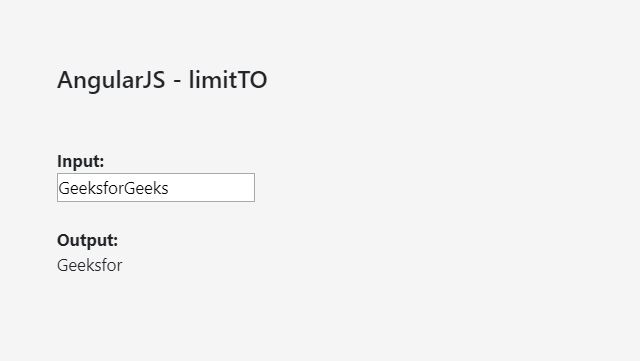Open in App
Not now

## Related Articles

• Write an Interview Experience

# AngularJS | limitTo Filter

• Last Updated : 09 May, 2019

The limitTo filter in AngularJS is used to returns an array or a string which contains a specified number of elements. This filter can be used with arrays, strings, and numbers. The basic principle, however, remains the same in all the three cases.

• For arrays, it returns an array containing only the specified number of items.
• When used for strings, it returns another string containing the specified number of characters.
• In the case of numbers, it returns a string containing only the specified number of digits.
• Negative numbers are used to return elements starting from the end of the element, instead of the beginning.

Syntax:

`{{ object | limitTo : limit : begin }}`

Parameters:

• limit: Number of returned elements.
• begin: Begin point of limitation. default is 0.

Example-1:

 ```<``html``>``<``script` `src``=``"https://ajax.googleapis.com/ajax/libs/angularjs/1.6.9/angular.min.js"``>``  ```` ` `<``body``>`` ` `    ``<``h2``>AngularJS - limitTO``    ``<``br``>``    ``<``br``>`` ` `    ``<``div` `ng-app``=``"myApp"` `ng-controller``=``"myCtrl"``>`` ` `        ``<``strong``>Input:``        ``<``br``>``        ``<``input` `type``=``"text"` `ng-model``=``"string"``>``        ``<``br``>``        ``<``br``>``        ``<``strong``>Output:``        ``<``br``>``        ``{{string|limitTo:4}}`` ` `    ```` ` `    ``<``script``>``        ``var app = angular.module('myApp', []);``        ``app.controller('myCtrl', function(\$scope) {``            ``\$scope.string = "";``        ``});``    ```` ` ``` ` ``

Output:Let’s have a look at another example to make things more clear.
Example-2:

 ```<``html``>``<``script` `src``=``"https://ajax.googleapis.com/ajax/libs/angularjs/1.6.9/angular.min.js"``>``  ```` ` `<``body``>`` ` `    ``<``h2``>AngularJS - limitTO``    ``<``br``>``    ``<``br``>`` ` `    ``<``div` `ng-app``=``"myApp"``         ``ng-controller``=``"myCtrl"``>`` ` `        ``<``strong``>Input:``        ``<``br``>``        ``<``input` `type``=``"text"``               ``ng-model``=``"firstName"``>``        ``<``br``>``        ``<``br``>``        ``<``strong``>Output:``        ``<``br``> {{firstName|limitTo:8}}`` ` `    ```` ` `    ``<``script``>``        ``var app = angular.module('myApp', []);``        ``app.controller('myCtrl', function(\$scope) {``            ``\$scope.firstName = "";``        ``});``    ```` ` ``` ` ``

Output:In this example, we can see that the limit in the ‘firstName’ expression has been specified to 8. Therefore it doesn’t matter how long the first name of the user is, only the first 8 characters of the first name will be displayed.

My Personal Notes arrow_drop_up